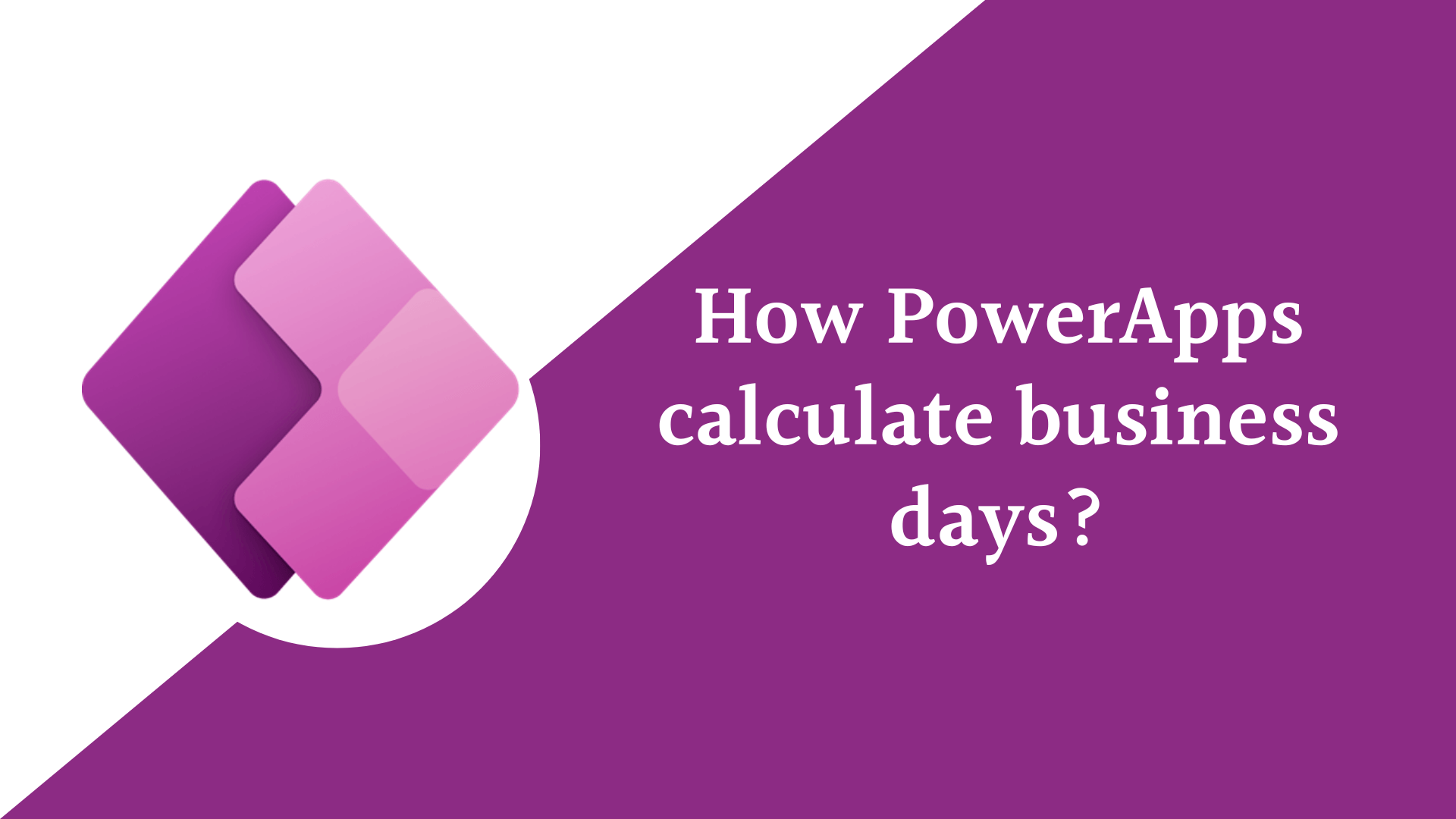## PowerApps Business Days Between Two Dates

• Post author:
• Post category:Power Apps

In this blog post we will learn How PowerApps Calculate business days between two dates excluding the Weekends and Holidays without using Power Automate.

## Calculate business days between two dates

The DateDiff function in PowerApps used to calculate the difference between two dates in a specified unit of time, the unit of time to use for the calculation. This can be one of the following: “day”, “hour”, “minute”, “second”, “month”, “quarter”, or “year”.

You can use this formula to calculate the business days between two dates and removing the weekends and holidays from these days.

``````RoundDown(DateDiff(DatePicker1.SelectedDate, DatePicker2.SelectedDate, TimeUnit.Days) / 7, 0) * 5 +
Mod(5 + Weekday(DatePicker2.SelectedDate) - Weekday(DatePicker1.SelectedDate), 5) -
CountIf(Holidays, StartDate >= DatePicker1.SelectedDate, StartDate <= DatePicker2.SelectedDate)``````

Let’s break down the formula to understand what this formula did.

1. `RoundDown(DateDiff(DatePicker1.SelectedDate, DatePicker2.SelectedDate, TimeUnit.Days) / 7, 0) * 5`
Calculates the number of full weeks between the selected dates, rounded down to the nearest whole number, and then multiplies it by 5 to get the total number of weekdays in those full weeks.
2. `Mod(5 + Weekday(DatePicker2.SelectedDate) - Weekday(DatePicker1.SelectedDate), 5)`
Calculates the number of weekdays between the two selected dates that are not part of a full week. The Power Apps `Weekday` function returns a number from 1 to 7 representing the day of the week, where 1 is Sunday and 7 is Saturday. This formula subtracts the weekday of the first selected date from the weekday of the second selected date, adds 5, and then takes the remainder when divided by 5 to get the number of weekdays in the partial week.
3. `CountIf(Holidays, StartDate >= DatePicker1.SelectedDate, StartDate <= DatePicker2.SelectedDate)`
Counts the number of holidays between the selected dates. The `CountIf` function counts the number of items in a collection that meet a certain condition , `Holidays` represent the name of your company holidays data source , you can save in excel, SharePoint , Collection, and so on.. In this case, it counts the number of items in the `Holidays` collection where the holiday falls between the two selected dates.
4. Finally, the formula subtracts the number of holidays from the total number of weekdays calculated in steps 1 and 2 to get the total number of business days between the two selected dates, taking into account weekends and holidays.

This is how to calculate business days between two dates in PowerApps.